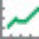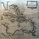623 vues
623
Rate of change of most volatile JPY and GBP pairs. All pairs ending in JPY are red except GBPJPY (colored yellow --currently most volatile 7/2016). GBPNZD is blue, the other GBP pairs are green, lime and teal. GBPJPY and GBPNZD are my favorite day trading / swing trading pairs. This script allows me to see the action of the most volatile and liquid pairs on one screen. JPY pairs (ex-GBPJPY) are all red so that I see the flow of JPY not so much each pair and its name. Global movement of JPY is what I am after. Same for the coloring of GBP pairs as green expect GBPNZD as blue. ***** EURGBP is plotted as an opposite (with a negative in front of its sma . EURGBP is extremely correlated to GBPNZD , I decided to plot it also.
```//@version=2
study(title="JPY GBP ROCs", shorttitle="JPY GBP ROCs")

l=input(defval=34)
s = input(title="Smoother length", type=integer,defval=12, minval=1)

p0 = input(title="Other data series", type=symbol,defval="FX_IDC:gbpaud")
p1 = input(title="Other data series", type=symbol,defval="FX_IDC:gbpnzd")
p2 = input(title="Other data series", type=symbol,defval="FX_IDC:gbptry")
p3 = input(title="Other data series", type=symbol,defval="FX_IDC:eurgbp")
p4 = input(title="Other data series", type=symbol,defval="FX_IDC:gbpcad")
p5 = input(title="Other data series", type=symbol,defval="FX_IDC:gbpchf")
p6 = input(title="Other data series", type=symbol,defval="FX_IDC:gbpjpy")
p7 = input(title="Other data series", type=symbol,defval="FX_IDC:gbpsgd")
p8 = input(title="Other data series", type=symbol,defval="FX_IDC:gbpusd")
p9 = input(title="Other data series", type=symbol,defval="FX_IDC:chfjpy")
p10 = input(title="Other data series", type=symbol,defval="FX_IDC:eurjpy")
p11 = input(title="Other data series", type=symbol,defval="FX_IDC:gbpnok")
p12 = input(title="Other data series", type=symbol,defval="FX_IDC:nzdjpy")
p13 = input(title="Other data series", type=symbol,defval="FX_IDC:audjpy")
p14 = input(title="Other data series", type=symbol,defval="FX_IDC:eurjpy")
p15 = input(title="Other data series", type=symbol,defval="FX_IDC:usdjpy")
p16 = input(title="Other data series", type=symbol,defval="FX_IDC:chfjpy")
s0= security(p0, period, close)
s1= security(p1, period, close)
s2= security(p2, period, close)
s3= security(p3, period, close)
s4= security(p4, period, close)
s5= security(p5, period, close)
s6= security(p6, period, close)
s7= security(p7, period, close)
s8= security(p8, period, close)
s9= security(p9, period, close)
s10= security(p10, period, close)
s11= security(p11, period, close)
s12= security(p12, period, close)
s13= security(p13, period, close)
s14= security(p14, period, close)
s15= security(p15, period, close)
s16= security(p16, period, close)
corr0 = correlation(close, s0, l)
corr1 = correlation(close, s1, l)
corr2 = correlation(close, s2, l)
corr3 = correlation(close, s3, l)
corr4 = correlation(close, s4, l)
corr5 = correlation(close, s5, l)
corr6 = correlation(close, s6, l)
corr7 = correlation(close, s7, l)
corr8 = correlation(close, s8, l)
corr9 = correlation(close, s9, l)
corr10 = correlation(close, s10, l)
corr11 = correlation(close, s11, l)
corr12 = correlation(close, s12, l)
corr13 = correlation(close, s13, l)
corr14 = correlation(close, s14, l)
corr15 = correlation(close, s15, l)
corr16 = correlation(close, s16, l)
r0 = roc(s0,l)
r1 = roc(s1,l)
r2 = roc(s2,l)
r3 = roc(s3,l)
r4 = roc(s4,l)
r5 = roc(s5,l)
r6 = roc(s6,l)
r7 = roc(s7,l)
r8 = roc(s8,l)
r9 = roc(s9,l)
r10 = roc(s10,l)
r11 = roc(s11,l)
r12 = roc(s12,l)
r13 = roc(s13,l)
r14 = roc(s14,l)
r15 = roc(s15,l)
r16 = roc(s16,l)

plot(sma(r7,s),color=lime,transp=0,title="GS",editable=true)
plot(sma(r0,s),color=green,transp=0,title="GA",editable=true)
plot(sma(r15,s),color=red,transp=0,title="UJ",editable=true)
plot(sma(r13,s),color=red,transp=0,title="AJ",editable=true)
plot(sma(r14,s),color=red,transp=0,title="EJ",editable=true)
plot(-sma(r3,s),color=aqua,transp=0,title="-EG",editable=true)
plot(sma(r1,s),color=blue,transp=0,title="GN",editable=true)
plot(sma(r6,s),color=yellow,transp=0,title="GJ",editable=true,linewidth=2)
plot(sma(r16,s),color=red,transp=0,title="CJ",editable=true)
plot(sma(r12,s),color=red,transp=0,title="NJ",editable=true)
hline(0,color=silver,linewidth=1,editable=true)
```Do you prefer this one to your Combined Slopes?
Répondrekakola
When I am doing day trading on 1-2min chart in the London-NY session I find this indicator better as it shows the ROCs individually. The one I actually use is this one but with only three or four ROCs on. GBPJPY GBPNZD and -EURGBP. The first two are the most volatile and I get in and out (hour or two) with 50-80 pips. For longer swing trading I use the combined slope on 15min chart. ** When day trading those high volatile ones I have been MOST successful trading in the direction of the larger HR and D chart trend and keeping an eye out for economic releases, they can turn your trade upside down. GBP has been dropping nicely for months which gives good odds of short positions.
Répondre